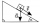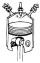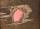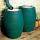# Pascal's law

Please calculate according to Pascal's law. Krupp's machines were known for their large size. In 1861, a blacksmith's steam hydraulic press was put into operation in Essen. What was the cross-sectional area of the larger piston if a compressive force of 150 kN could develop by applying a force of 200N to a small piston with a capacity of 40 cm2?

S2 =  30000 cm2

### Step-by-step explanation:Did you find an error or inaccuracy? Feel free to write us. Thank you!## Related math problems and questions:What overload in g (g-force) has passed the pilot if he accelerated from 0 to 600 km/h in 3 seconds?
• Two rectanglesI cut out two rectangles with 54 cm², 90 cm². Their sides are expressed in whole centimeters. If I put these rectangles together I get a rectangle with an area of 144 cm2. What dimensions can this large rectangle have? Write all options. Explain your calc
• Heat transferWe placed a lead object weighing 0.4 kg and 250°C in 0.4 L water. What was the initial temperature of water t2 if the object's temperature and the water after reaching equilibrium was 35°C? We assume that the heat exchange occurred only between the lead o
• ResistanceDetermine the resistance of the bulb with the current 200 mA and is in a regular lamp (230V).
• Inclined planeThe body stays on an inclined plane and exerts a compressive force of 70N on it. Find the angle between the inclined plane and the horizontal if a gravitational force of 100N acts on the body.
• The workThe work was to be done by 150 workers. At the beginning of their work, their number reduced by 40, which increased the time of work by 5 and 1/3 of the schedule. How long did work take?
• Total displacementCalculate total displacement of the 4-cylinder engine with the diameter of the piston bore B = 6.6 cm and stroke S=2.4 cm of the piston. Help: the crankshaft makes one revolution while the piston moves from the top of the cylinder to the bottom and back t
• CranberriesThey brought 65 kg of dried cranberries to the packing house. They made 150-gram and 200-gram packages from them. How many kilograms of cranberries did they use for the smaller 200 packages? How many 200 gram packages were there?
• MegapascalsWhat is the area of crosssection of the piston, if the force of 300 kN produces a pressure of 5 MPa?
• ShotShot with a mass 43 g flying at 256 m/s penetrates into the wood to a depth 25 cm. What is the average force of resistance of wood?
• Map scaleGarden has on plan on a scale of 1: 150 width 22 cm and length 35 cm. What is the real area of the garden?
• Electric workCalculate the work done by the electric forces passing the current of 0.2 A through the bulb in 10 minutes if the bulb is connected to a 230 V power supply.
• Basic formExpressed the ratios of values in the basic form: 0,5 t : 1,2 kg 200 l : 0,15 m3 12 t : 3600 kg 500 kg : 2,5 t 0,9 kg : 500 g 3,6 m : 240 cm 1200 mm : 2,4 m 300 l : 0,3 m3 6 min 30 s : 900 s
• Electric input powerSolve problems related to electric power: a) U = 120 V, I = 0.5 A, P =? b) P = 200 W, U = 230 V, I =? c) I = 5 A, P = 2200W, U =?
• Two numbersWe have two numbers. Their sum is 140. One-fifth of the first number is equal to half the second number. Determine those unknown numbers.
• Inflow - outflowThe tank will fill by inflow for 143 minutes and empties by outflow for 150 minutes. How long take to fill tank if it is also opened the inflow and outflow?
• TransformerSolve the textbook problems - transformer: a) N1 = 40, N2 = 80, U2 = 80 V, U1 =? b) N1 = 400, U1 = 200 V, U2 = 50 V, N2 =?